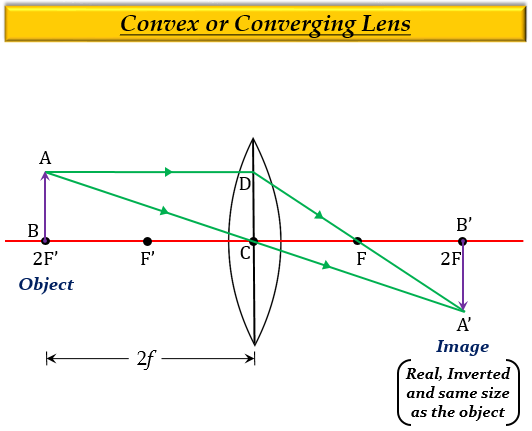# A 1 cm high object is placed at a distance of 2f from a convex lens. What is the height of the image formed?

A 1 cm high object is placed at a distance of 2f from a convex lens, then the height of the image formed will also be 1 cm because when an object is placed at a distance of $2f$ from a convex lens, then the size of the image formed is equal to the size of the object.

Explanation

When an object is at $(2F')$ of a convex lens, which means the object is at a distance equal to twice the focal length, $2f$ of a convex lens, the image formed is real, inverted, same size as the object, and at a distance $2f$ on the other side of the lens.Updated on: 10-Oct-2022

2K+ Views